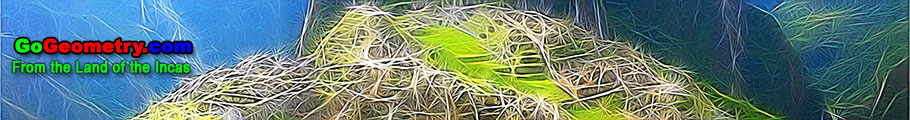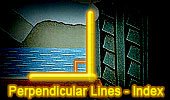Perpendicular Lines, Theorems and Problems - Table of Content (3)Geometry Problem 1200 Square, Right Triangle, Perpendicular, 90 Degrees, Equal Areas. Geometry Problem 1198 Right Triangle, Square, Rectangle, Quadrilateral, Altitude, Equal Areas. Geometry Problem 1194 Equilateral Triangle, Perpendicular, Distance, Side, Metric Relations. Geometry Problem 1180 Quadrilateral, 120 Degrees, Diagonals, Perpendicular. Geometry Problem 1169 Complete Quadrilateral, Orthocenters of the Component Triangles, Collinear Points, Ortholine, Steiner-line, Orthocentric line. Geometry Problem 1167. Triangle, Circle, Circumcircle, Perpendicular, 90 Degrees, Collinear Points. Geometry Problem 1165. Right Triangle, Circle, Center, Radius, Cathetus, Perpendicular, 90 Degrees, Perimeter, Metric Relations. Geometry Problem 1163. Isosceles Triangle, Congruence, Double Angle, Circle, Concyclic Points, Circumcenter, Perpendicular, Angle Bisector. Geometry Problem 1157. Four Circles, Mutually Tangent, 90 Degrees, Radius, Metric Relations. Go to Page: Previous | 1 | 2 | 3 | 4 | 5 | 6 | 7 | 8 | 9 | 10 | 11 | 12 | 13 | 14 | 15 | 16 | 17 | 18 | 19 | 20 | Next

 Home | Search | Geometry | Post a comment | Email | Post a comment | By Antonio Gutierrez Last updated: Feb 15, 2015